Chapter 14. Oxidation and Reduction

# Balancing Redox Reactions

Learning Objectives

1. Learn to balanced simple redox reactions by inspection.
2. Learn to balance complex redox reactions by the half reaction method.
3. Use the solvent, or parts of it, as a reactant or a product in balancing a redox reaction.

Balancing simple redox reactions can be a straightforward matter of going back and forth between products and reactants. For example, in the redox reaction of Na and Cl2:

Na + Cl2 → NaCl

it should be immediately clear that the Cl atoms are not balanced. We can fix this by putting the coefficient 2 in front of the product:

Na + Cl2 → 2NaCl

However, now the sodium is unbalanced. This can be fixed by including the coefficient 2 in front of the Na reactant:

2Na + Cl2 → 2NaCl

This reaction is now balanced. That was fairly straightforward; we say that we are able to balance the reaction by inspection. Many simple redox reactions can be balanced by inspection.

Example 14.3

Balance this redox reaction by inspection.

SO2 + O2 → SO3

Solution
There is one S atom on both sides of the equation, so the sulfur is balanced. However, the reactant side has four O atoms while the product side has three. Clearly we need more O atoms on the product side, so let us start by including the coefficient 2 on the SO3:

SO2 + O2 → 2SO3

This now gives us six O atoms on the product side, and it also imbalances the S atoms. We can balance both the elements by adding coefficient 2 on the SO2 on the reactant side:

2SO2 + O2 → 2SO3

This gives us two S atoms on both sides and a total of six O atoms on both sides of the chemical equation. This redox reaction is now balanced.

Test Yourself
Balance this redox reaction by inspection.

Al + O2 → Al2O3

4Al + 3 O2 → 2Al2O3

The first thing you should do when encountering an unbalanced redox reaction is to try to balance it by inspection.

Some redox reactions are not easily balanced by inspection. Consider this redox reaction:

Al + Ag+ → Al3+ + Ag

At first glance, this equation seems balanced: there is one Ag atom on both sides and one Al atom on both sides. However, if you look at the total charge on each side, there is a charge imbalance: the reactant side has a total charge of 1+, while the product side has a total charge of 3+. Something is amiss with this chemical equation; despite the equal number of atoms on each side, it is not balanced.

A fundamental point about redox reactions that has not arisen previously is that the total number of electrons being lost must equal the total number of electrons being gained for a redox reaction to be balanced. This is not the case for the aluminum and silver reaction: the Al atom loses three electrons to become the Al3+ ion, while the Ag+ ion gains only one electron to become elemental silver.

To balance this, we will write each oxidation and reduction reaction separately, listing the number of electrons explicitly in each. Individually, the oxidation and reduction reactions are called half reactions. We will then take multiples of each reaction until the number of electrons on each side cancels completely and combine the half reactions into an overall reaction, which should then be balanced. This method of balancing redox reactions is called the half reaction method. (There are other ways of balancing redox reactions, but this is the only one that will be used in this text. The reason for this will be seen in the section “Applications of Redox Reactions: Voltaic Cells”.)

The oxidation half reaction involves aluminum, which is being oxidized:

Al → Al3+

This half reaction is not completely balanced because the overall charges on each side are not equal. When an Al atom is oxidized to Al3+, it loses three electrons. We can write these electrons explicitly as products:

Al → Al3+ + 3e

Now this half reaction is balanced — in terms of both atoms and charges.

The reduction half reaction involves silver:

Ag+ → Ag

The overall charge is not balanced on both sides. But we can fix this by adding one electron to the reactant side because the Ag+ ion must accept one electron to become the neutral Ag atom:

Ag+ + e → Ag

This half reaction is now also balanced.

When combining the two half reactions into a balanced chemical equation, the key is that the total number of electrons must cancel, so the number of electrons lost by atoms are equal to the number of electrons gained by other atoms. This may require we multiply one or both half reaction(s) by an integer to make the number of electrons on each side equal. With three electrons as products and one as reactant, the least common multiple of these two numbers is three: we can use a single aluminum reaction but must take three times the silver reaction: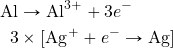The 3 on the second reaction is distributed to all species in the reaction: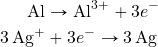Now the two half reactions can be combined just like two algebraic equations, with the arrow serving as the equals sign. The same species on opposite sides of the arrow can be cancelled: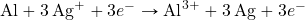The net balanced redox reaction is as follows: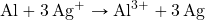There is still only one Al atom on each side of the chemical equation, but there are now three Ag atoms, and the total charge on each side of the equation is the same (3+ for both sides). This redox reaction is balanced. It took more effort to use the half reaction method than by inspection, but the correct balanced redox reaction was obtained.

Example 14.4

Balance this redox reaction by using the half reaction method.

Fe2+ + Cr → Fe + Cr3+

Solution
We start by writing the two half reactions. Chromium is being oxidized, and iron is being reduced: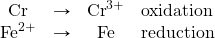Then we include the appropriate number of electrons on the proper side to balance the charges for each reaction: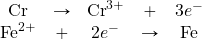The first reaction involves three electrons, while the second reaction involves two electrons. The least common multiple of these two numbers is six, so to get six electrons in each reaction we need to double the first reaction and triple the second one: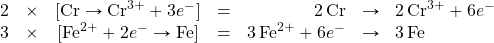We can combine the two final reactions, noting that the electrons cancel: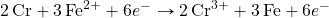The overall, balanced redox reaction is: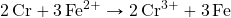Test Yourself
Balance this redox reaction by using the half reaction method.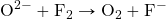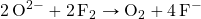Many redox reactions occur in aqueous solution — in water. Because of this, in many cases H2O or a fragment of an H2O molecule (H+ or OH, in particular) can participate in the redox reaction. As such, we need to learn how to incorporate the solvent into a balanced redox equation.

Consider the following oxidation half reaction in aqueous solution, which has one Cr atom on each side:

Cr3+ → CrO4

Here, the Cr atom is going from the +3 to the +7 oxidation state. To do this, the Cr atom must lose four electrons. Let us start by listing the four electrons as products:

Cr3+ → CrO4 + 4e

But where do the O atoms come from? They come from water molecules or a common fragment of a water molecule that contains an O atom: the OH ion. When we balance this half reaction, we should feel free to include either of these species in the reaction to balance the elements. Let us use H2O to balance the O atoms; we need to include four water molecules to balance the four O atoms in the products:

4H2O + Cr3+ → CrO4 + 4e

This balances the O atoms, but now introduces hydrogen to the reaction. We can balance the H atoms by adding an H+ ion, which is another fragment of the water molecule. We need to add eight H+ ions to the product side:

4H2O + Cr3+ → CrO4 + 4e + 8H+

The Cr atoms are balanced, the O atoms are balanced, and the H atoms are balanced; if we check the total charge on both sides of the chemical equation, they are the same (3+, in this case). This half reaction is now balanced, using water molecules and parts of water molecules as reactants and products.

Reduction reactions can be balanced in a similar fashion. When oxidation and reduction half reactions are individually balanced, they can be combined in the same fashion as before: by taking multiples of each half reaction as necessary to cancel all electrons. Other species, such as H+, OH, and H2O, may also have to be cancelled in the final balanced reaction.

Unless otherwise noted, it does not matter if you add H2O or OH as a source of O atoms, although a reaction may specify acidic solution or basic solution as a hint of what species to use or what species to avoid. OH ions are not very common in acidic solutions, so they should be avoided in those circumstances.

Example 14.5

Balance this redox reaction. Assume a basic solution.

MnO2 + CrO3 → Mn + CrO4

Solution
We start by separating the oxidation and reduction processes so we can balance each half reaction separately. The oxidation reaction is as follows:

CrO3 → CrO4

The Cr atom is going from a +5 to a +7 oxidation state and loses two electrons in the process. We add those two electrons to the product side:

CrO3 → CrO4 + 2e

Now we must balance the O atoms. Because the solution is basic, we should use OH rather than H2O:

OH + CrO3 → CrO4 + 2e

We have introduced H atoms as part of the reactants; we can balance them by adding H+ as products:

OH + CrO3 → CrO4 + 2e + H+

If we check the atoms and the overall charge on both sides, we see that this reaction is balanced. However, if the reaction is occurring in a basic solution, it is unlikely that H+ ions will be present in quantity. The way to address this is to add an additional OH ion to each side of the equation:

OH + CrO3 + OH → CrO4 + 2e + H+ + OH

The two OH ions on the left side can be grouped together as 2OH. On the right side, the H+ and OH ions can be grouped into an H2O molecule:

2OH + CrO3 → CrO4 + 2e + H2O

This is a more appropriate form for a basic solution.

Now we balance the reduction reaction:

MnO2 → Mn

The Mn atom is going from +4 to 0 in oxidation number, which requires a gain of four electrons:

4e + MnO2 → Mn

Then we balance the O atoms and then the H atoms:

4e + MnO2 → Mn + 2 OH
2 H+ + 4e + MnO2 → Mn + 2 OH

We add two OH ions to each side to eliminate the H+ ion in the reactants; the reactant species combine to make two water molecules, and the number of OH ions in the product increases to four:

2 H2O + 4e + MnO2 → Mn + 4 OH

This reaction is balanced for a basic solution.

Now we combine the two balanced half reactions. The oxidation reaction has two electrons, while the reduction reaction has four. The least common multiple of these two numbers is four, so we multiply the oxidation reaction by 2 so that the electrons are balanced:

2 × [2OH + CrO3 → CrO4 + 2e + H2O]
2H2O + 4e + MnO2 → Mn + 4 OH

Combining these two equations results in the following equation:

4 OH + 2CrO3 + 2H2O + 4e + MnO2 → 2CrO4 + 4e + 2H2O + Mn + 4OH

The four electrons cancel. So do the two H2O molecules and the four OH ions. What remains is

2CrO3 + MnO2 → 2CrO4 + Mn

which is our final balanced redox reaction.

Test Yourself
Balance this redox reaction. Assume a basic solution.

Cl + MnO4 → MnO2 + ClO3

H2O + Cl + 2MnO4 → 2MnO2 + ClO3 + 2OH

Key Takeaways

• Redox reactions can be balanced by inspection or by the half reaction method.
• A solvent may participate in redox reactions; in aqueous solutions, H2O, H+, and OH may be reactants or products.

Exercises

# Questions

1. Balance these redox reactions by inspection.
1. Na + F2 → NaF
2. Al2O3 + H2 → Al + H2O
2. Balance these redox reactions by inspection.
1. Fe2S3 + O2 → Fe2O3 + S
2. Cu2O + H2 → Cu + H2O
3. Balance these redox reactions by inspection.
1. CH4 + O2 → CO2 + H2O
2. P2O5 + Cl2 → PCl3 + O2
4. Balance these redox reactions by inspection.
1. PbCl2 + FeCl3 → PbCl4 + FeCl2
2. SO2 + F2 → SF4 + OF2
5. Balance these redox reactions by the half reaction method.
1. Ca + H+ → Ca2+ + H2
2. Sn2+ → Sn + Sn4+ (Hint: both half reactions will start with the same reactant.)
6. Balance these redox reactions by the half reaction method.
1. Fe3+ + Sn2+ → Fe + Sn4+
2. Pb2+ → Pb + Pb4+ (Hint: both half reactions will start with the same reactant.)
7. Balance these redox reactions by the half reaction method.
1. Na + Hg2Cl2 → NaCl + Hg
2. Al2O3 + C → Al + CO2
8. Balance these redox reactions by the half reaction method.
1. Br + I2 → I + Br2
2. CrCl3 + F2 → CrF3 + Cl2
9. Balance these redox reactions that occur in aqueous solution. Use whatever water-derived species is necessary; there may be more than one correct balanced equation.
1. Cu + NO3 → Cu2+ + NO2
2. Fe + MnO4 → Fe3+ + Mn
10. Balance these redox reactions that occur in aqueous solution. Use whatever water-derived species is necessary; there may be more than one correct balanced equation.
1. CrO3 + Ni2+ → Cr3+ + Ni3+
2. OsO4 + C2H4 → Os + CO2
11. Balance these redox reactions that occur in aqueous solution. Use whatever water-derived species is necessary; there may be more than one correct balanced equation.
1. ClO + Ti2+ → Ti4+ + Cl
2. BrO3 + Ag → Ag+ + BrO2
12. Balance these redox reactions that occur in aqueous solution. Use whatever water-derived species is necessary; there may be more than one correct balanced equation.
1. H2O2 + NO → N2O3 + H2O
2. VO2+ + NO → V3+ + NO2
13. Explain why this chemical equation is not balanced and balance it if it can be balanced.

Cr2+ + Cl2 → Cr3+ + 2Cl

14. Explain why this equation is not balanced and balance it if it can be balanced.

O2 + 2H2O + Br2 → 4OH + 2Br

1. 2Na + F2 → 2NaF
2. Al2O3 + 3H2 → 2Al + 3H2O
1. CH4 + 2O2 → CO2 + 2H2O
2. 2P2O5 + 6Cl2 → 4PCl3 + 5O2
1. Ca + 2H+ → Ca2+ + H2
2. 2Sn2+ → Sn + Sn4+
1. 2Na + Hg2Cl2 → 2NaCl + 2Hg
2. 2Al2O3 + 3C → 4Al + 3CO2
1. 4H+ + Cu + 2NO3 → Cu2+ + 2NO2 + 2H2O in acidic solution; 2H2O + Cu + 2NO3 → Cu2+ + 2NO2 + 4OH in basic solution
2. 24H+ + 3MnO4 + 7Fe → 7Fe3+ + 3Mn + 12H2O in acidic solution; 12H2O + 3MnO4 + 7Fe → 7Fe3+ + 3Mn + 24OH in basic solution
1. 2H+ + ClO + Ti2+ → Cl + H2O + Ti4+ in acidic solution; H2O + ClO + Ti2+ → Cl + Ti4+ + 2OH in basic solution
2. 2H+ + BrO3 + Ag → BrO2 + H2O + Ag+ in acidic solution; H2O + BrO3 + Ag → BrO2 + Ag+ + 2OH in basic solution
1. The charges are not properly balanced. The correct balanced equation is 2Cr2+ + Cl2 → 2Cr3+ + 2Cl.